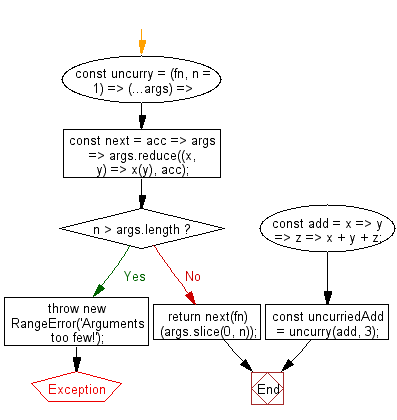# JavaScript: Uncurry a function up to depth n

## JavaScript fundamental (ES6 Syntax): Exercise-114 with Solution

Write a JavaScript program to uncurry a function up to depth n.

• Use Array.prototype.reduce() on the provided arguments to call each subsequent curry level of the function.
• If the length of the provided arguments is less than n throw an error.
• Otherwise, call fn with the proper amount of arguments, using Array.prototype.slice(0, n).
• Omit the second argument, n, to uncurry up to depth 1.

Sample Solution:

JavaScript Code:

``````//#Source https://bit.ly/2neWfJ2
const uncurry = (fn, n = 1) => (...args) => {
const next = acc => args => args.reduce((x, y) => x(y), acc);
if (n > args.length) throw new RangeError('Arguments too few!');
return next(fn)(args.slice(0, n));
};
const add = x => y => z => x + y + z;
```
```

Sample Output:

```6
```

Pictorial Presentation:Flowchart:Live Demo:

See the Pen javascript-basic-exercise-114-1 by w3resource (@w3resource) on CodePen.

Improve this sample solution and post your code through Disqus

What is the difficulty level of this exercise?

Test your Programming skills with w3resource's quiz.

﻿

## JavaScript: Tips of the Day

Chunks an array into n smaller arrays

Example:

```const tips_chunkIntoN = (arr, n) => {
const size = Math.ceil(arr.length / n);
return Array.from({ length: n }, (v, i) =>
arr.slice(i * size, i * size + size)
);
}
console.log(tips_chunkIntoN([1, 2, 3, 4, 5, 6, 7,8], 4));
```

Output:

```[[1,2],[3,4],[5,6],[7,8]]
```